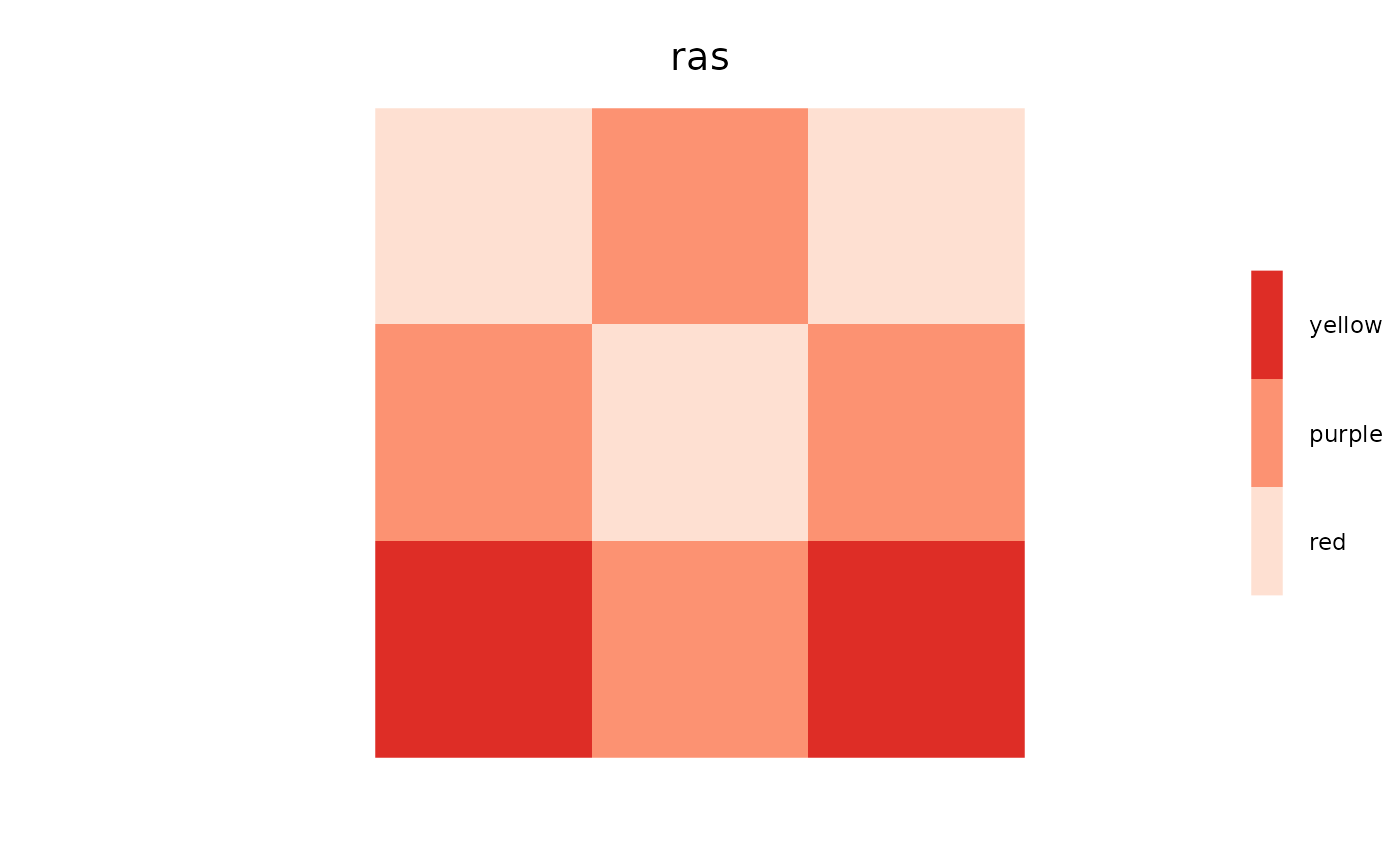Get and set colours for plotting Raster* objects

setColors works as a replacement method or a normal function call. This function can accept RColorBrewer colours by name. See examples.

getColors(object)

# S4 method for Raster
getColors(object)

# S4 method for ANY
getColors(object)

# S4 method for SpatialPoints
getColors(object)

setColors(object, ..., n) <- value

# S4 method for RasterLayer,numeric,character
setColors(object, ..., n) <- value

# S4 method for RasterLayer,missing,character
setColors(object, ..., n) <- value

# S4 method for RasterStack,numeric,list
setColors(object, ..., n) <- value

# S4 method for Raster,missing,list
setColors(object, ..., n) <- value

setColors(object, value, n)

# S4 method for RasterLayer,character,numeric
setColors(object, value, n)

# S4 method for RasterLayer,character,missing
setColors(object, value)

## Arguments

object

A Raster* object.

...

Additional arguments to colorRampPalette.

n

An optional vector of values specifying the number of levels from which to interpolate the colour palette.

value

Named list of hex colour codes (e.g., from RColorBrewer::brewer.pal), corresponding to the names of RasterLayers in x.

## Value

Returns a named list of colours.

Returns a Raster with the colortable slot set to values.

setColors<-(), brewer.pal()

brewer.pal(), colorRampPalette().

Alex Chubaty

## Examples

library(igraph)
library(raster)

ras <- raster(matrix(c(0, 0, 1, 2), ncol = 2, nrow = 2))

getColors(ras) ## none
#> $layer #> character(0) #> # Use replacement method setColors(ras, n = 3) <- c("red", "blue", "green") getColors(ras) #>$layer
#>  "#FF0000FF" "#0000FFFF" "#00FF00FF"
#>

clearPlot()
Plot(ras)# Use function method
ras <- setColors(ras, n = 3, c("red", "blue", "yellow"))
getColors(ras)
#> $layer #>  "#FF0000FF" "#0000FFFF" "#FFFF00FF" #> clearPlot() Plot(ras)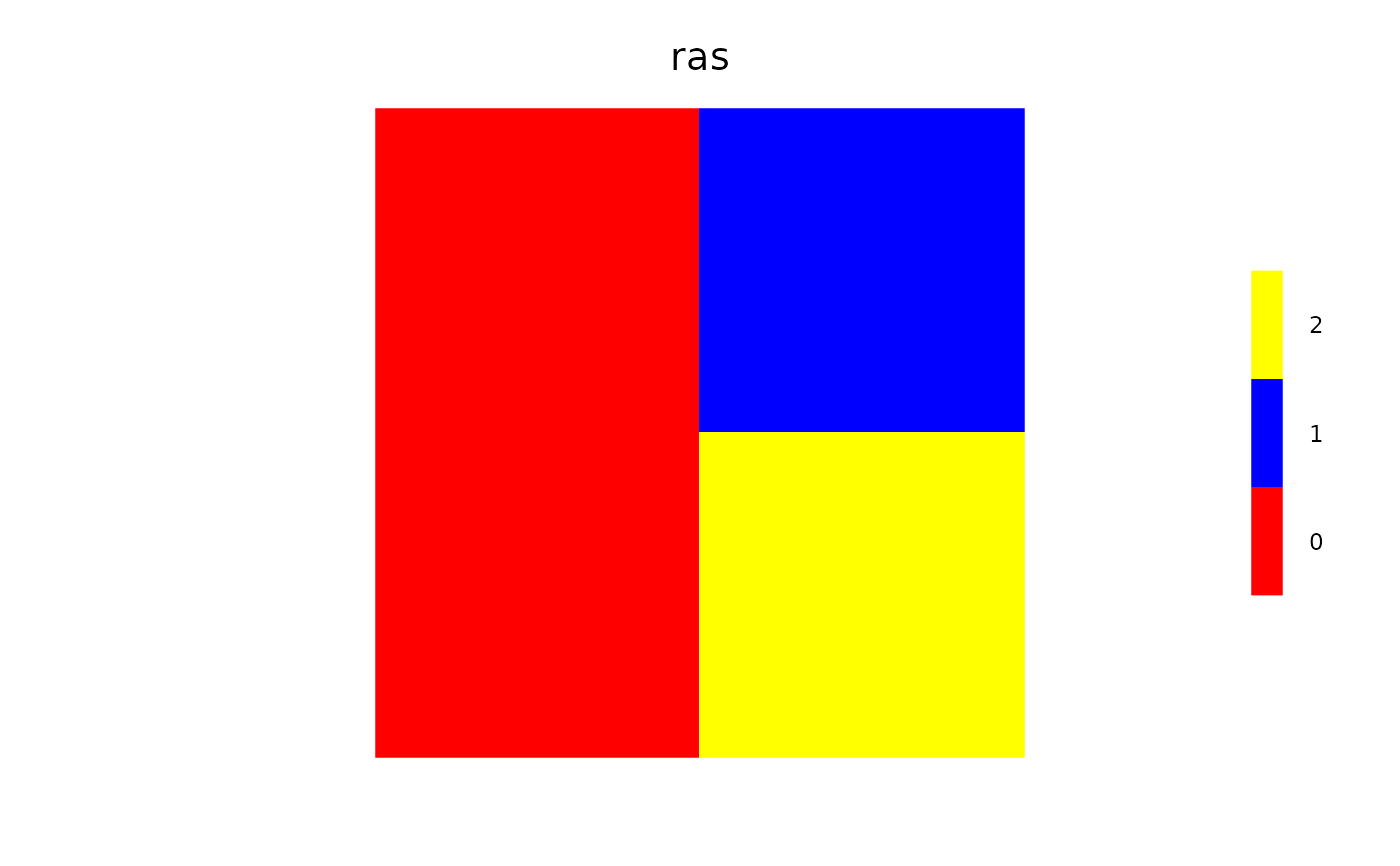# Using the wrong number of colors, e.g., here 2 provided, # for a raster with 3 values... causes interpolation, which may be surprising ras <- setColors(ras, c("red", "blue")) clearPlot() Plot(ras)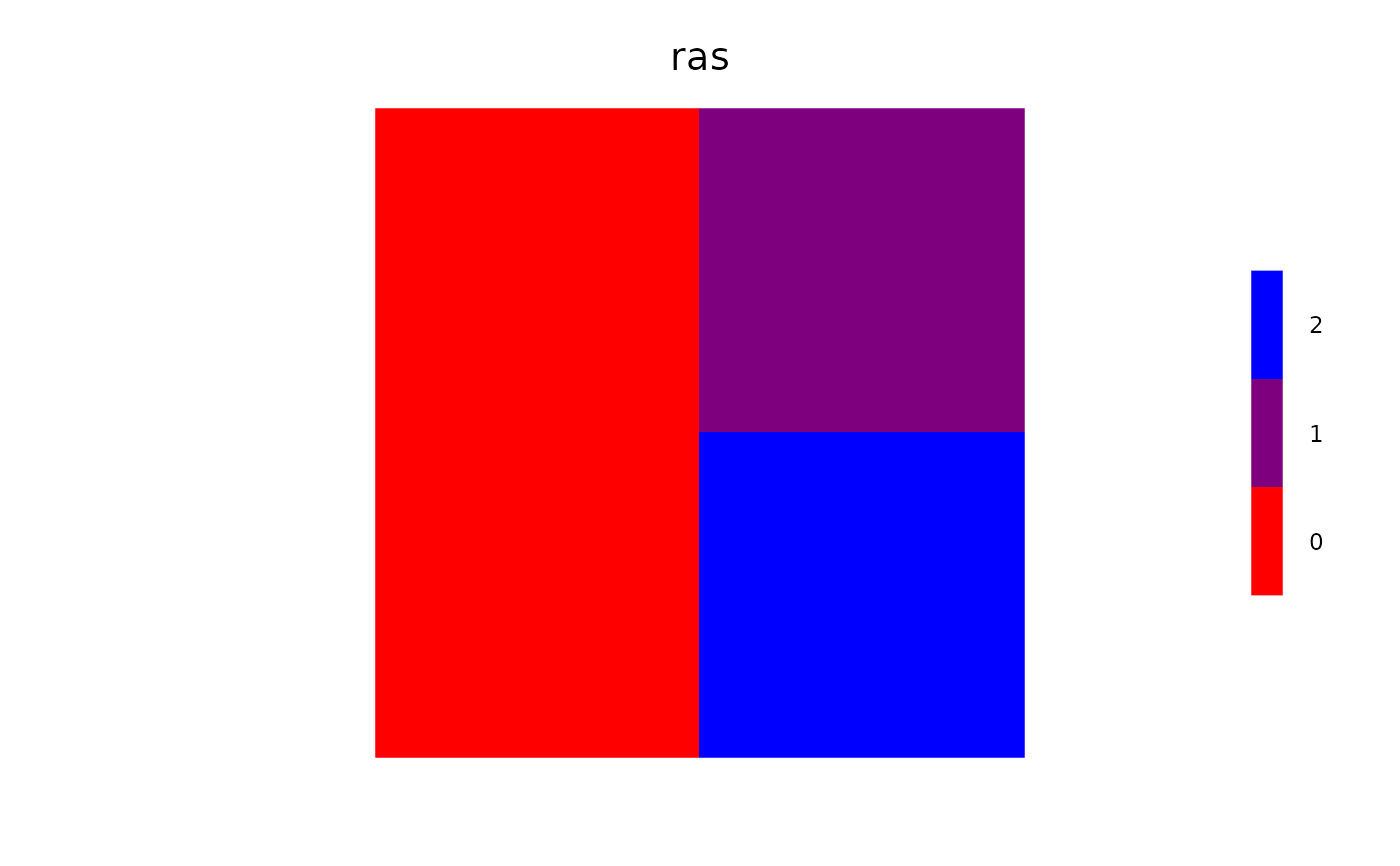# Real number rasters - interpolation is used ras <- raster(matrix(runif(9), ncol = 3, nrow = 3)) %>% setColors(c("red", "yellow")) # interpolates when real numbers, gives warning clearPlot() Plot(ras)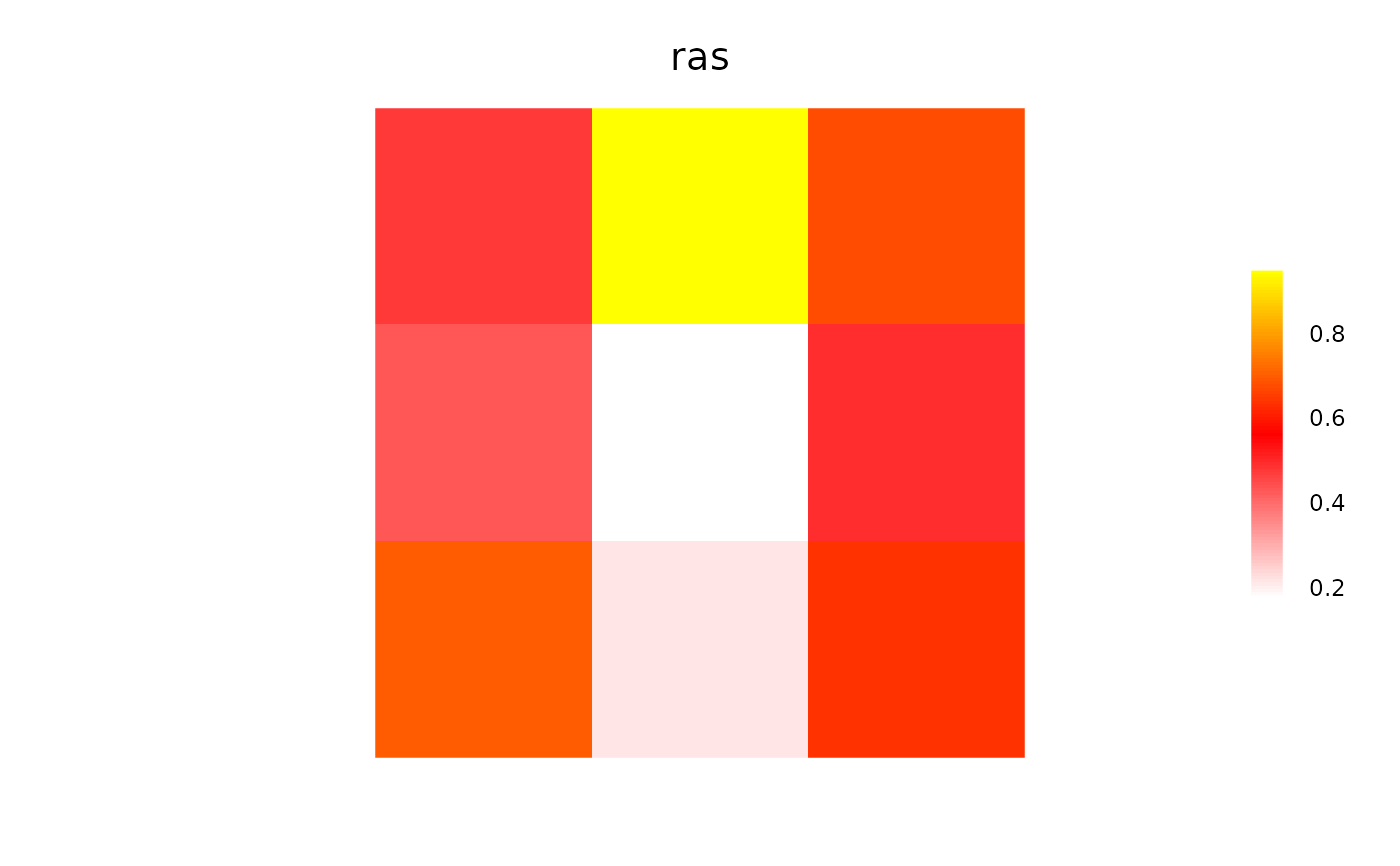# Factor rasters, can be contiguous (numerically) or not, in this case not: ras <- raster(matrix(sample(c(1, 3, 6), size = 9, replace = TRUE), ncol = 3, nrow = 3)) levels(ras) <- data.frame(ID = c(1, 3, 6), Names = c("red", "purple", "yellow")) ras <- setColors(ras, n = 3, c("red", "purple", "yellow")) getColors(ras) #>$layer
#>  "red"    "purple" "yellow"
#>

clearPlot()
Plot(ras)# if a factor raster, and not enough labels are provided, then a warning
#   will be given, and colors will be interpolated
#   The level called purple is not purple, but interpolated betwen red and yellow
suppressWarnings({
ras <- setColors(ras, c("red", "yellow"))
clearPlot()
Plot(ras)
})
#> Number of colours not equal number of values: interpolating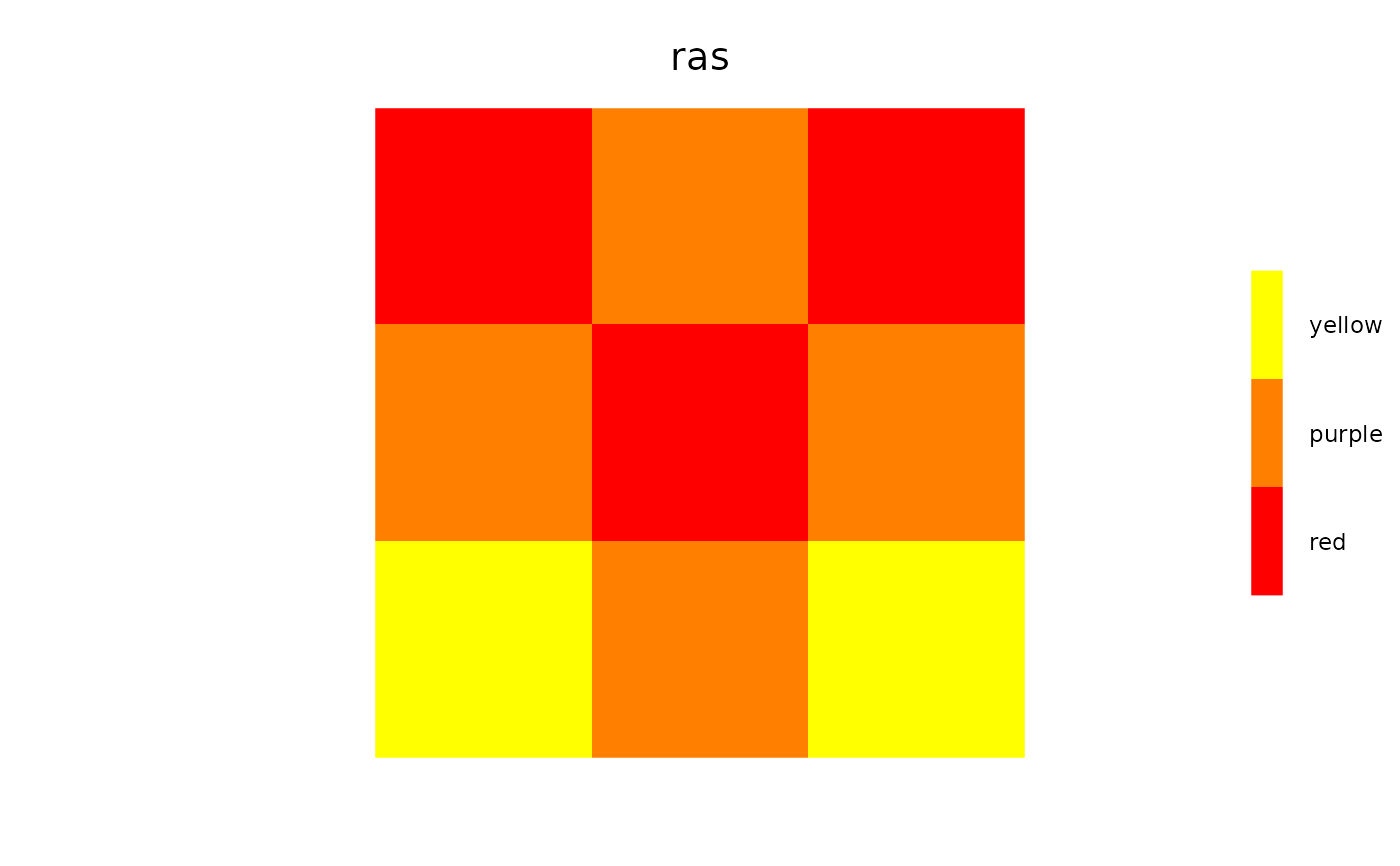# use RColorBrewer colors
setColors(ras) <- "Reds"
#> Number of colours not equal number of values: interpolating
clearPlot()
Plot(ras)